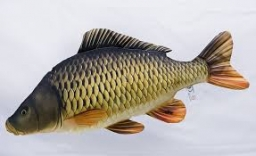# Competition 8193

David caught three carp races. The second was 15 cm longer than the first. The third is 8 cm shorter than the second. David entered a total length of 166 cm in the competition. How big a carp did he catch?

a =  48
b =  63
c =  55

### Step-by-step explanation:

b=15+a
c = b-8
a+b+c = 166

a-b = -15
b-c = 8
a+b+c = 166

Row 3 - Row 1 → Row 3
a-b = -15
b-c = 8
2b+c = 181

Pivot: Row 2 ↔ Row 3
a-b = -15
2b+c = 181
b-c = 8

Row 3 - 1/2 · Row 2 → Row 3
a-b = -15
2b+c = 181
-1.5c = -82.5

c = -82.5/-1.5 = 55
b = 181-c/2 = 181-55/2 = 63
a = -15+b = -15+63 = 48

a = 48
b = 63
c = 55

Our linear equations calculator calculates it.Did you find an error or inaccuracy? Feel free to write us. Thank you!

Tips for related online calculators
Do you have a linear equation or system of equations and looking for its solution? Or do you have a quadratic equation?
Do you want to convert length units?